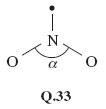Science, Maths & Technology

### Become an OU student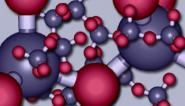The molecular world

Start this free course now. Just create an account and sign in. Enrol and complete the course for a free statement of participation or digital badge if available.

# 6.4 Summary of Section 6

1. Molecules have a three-dimensional shape. Bulky irregularities in the shape of a molecule around a reactive site can exclude a potential reactant. Such effects are described as steric.

2. A sufficient refinement of the molecular shape in the region of the reactive site can make that site specific to just one particular reactant. Many enzymes operate in this way.

3. The shapes of simple molecules can be predicted using valence-shell electron-pair repulsion theory. The valence electrons of a central atom are divided between the bonds to other atoms, and non-bonded pairs, each bond or non-bonded pair constituting a repulsion axis. The total number of repulsion axes determines their arrangement in space (see Figure 47): two, linear; three, trigonal planar; four, tetrahedral; five, trigonal bipyramidal; six, octahedral; seven, pentagonal bipyramidal.

4. A choice between alternative distributions of bonds and non-bonded pairs within any one of these arrangements can usually be made by minimising the strongest types of repulsion. In making this choice, the following points are relevant:

• (i) Repulsive effects involving non-bonded pairs and bond pairs vary in the order: non-bonded pair-non-bonded pair > non-bonded pair-bond pair > bond pair-bond pair

• (ii) Multiple bonds exert stronger repulsions than single bonds.

• (iii) In a trigonal-bipyramidal distribution of repulsion axes, non-bonded pairs occupy equatorial rather than axial positions.

## Question 17

The six carbon atoms of benzene, C6H6, lie at the corners of a regular hexagon, and each one carries a hydrogen atom. In compounds 7.13-7.16 below, some of these hydrogen atoms have been replaced by other groups. In each case, a carboxylic acid functional group, —﻿COOH, is present.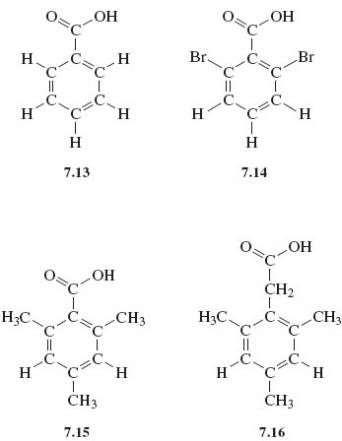The —COOH group is normally converted to —COOCH3 when a compound containing it is heated in a solution containing hydrogen chloride and methanol, CH3OH. When compounds 7.13-7.16 are subjected to this treatment, 7.13 and 7.16 react as expected, forming C6H5COOCH3 and (CH3)﻿3C6H2CH2COOCH3, respectively. But compounds 7.14 and 7.15 undergo little or no reaction. Explain these differences.

We shall number the carbon atoms of the benzene ring from 1 to 6, calling the atom to which the —COOH or —CH2COOH units are attached, carbon number 1. In Structure 7.13, hydrogen atoms (relatively small) are attached to positions 2 and 6. Incoming reactants attacking the —COOH group attached to carbon 1 are therefore relatively unimpeded, and reaction occurs readily. In Structures 7.14 and 7.15, the 2 and 6 positions are occupied by the much more bulky —Br and —CH3 groups, respectively. These impede access of the incoming reactant to the —COOH group, thereby hindering the reaction. In Structure 7.16, the insertion of a —CH2— unit between the —COOH group and carbon 1 increases the distance between the —CH3 groups in positions 2 and 6 and the —COOH group. Steric hindrance is no longer severe and reaction once more occurs readily.

## Question 18

By heating the solids BeCl2 and SnCl2 to quite moderate temperatures, discrete gaseous BeCl2 and SnCl2 molecules can be obtained. What shapes and bond angles would you expect the molecules to have?

Beryllium has two valence electrons. Both are used up in forming the two bonds to chlorine. There are two repulsion axes, so BeCl2 should be linear; this is the case. Tin has four valence electrons. Two are used in forming the two single bonds to chlorine, and this leaves one non-bonded pair. There are three repulsion axes, which should therefore be disposed in a triangular sense (see Structure Q.27), so SnCl2 should be V-shaped with a bond angle slightly less than 120° because of the primacy of the non-bonded pair-bond pair repulsions. Experimentally, this is found to be so.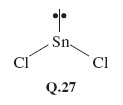## Question 19

Predict the shapes of the ions (i) NH4+; (ii) ICl2− (central atom I); (iii) PCl6− (central atom P).

• (i) Nitrogen has five valence electrons, but as the NH4+ ion carries a positive charge, one must be subtracted, leaving four. All four electrons are used to form the four bonds to hydrogen, so there are just four repulsion axes, and the ion has a tetrahedral shape.

• (ii) Iodine has seven valence electrons, and if one electron is added for the single negative charge on ICl2, this becomes eight. Two of the eight electrons are used in forming single bonds to the two chlorines, leaving six which are divided into three non-bonded pairs. There are therefore five repulsion axes, which take on a trigonal-bipyramidal disposition (cf. PF5, Figure 47d). In this arrangement, non-bonded pairs occupy equatorial positions leaving the axial positions for the two chlorines. Thus, ICl2 is linear (Structure Q.28).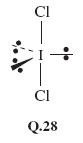• (iii) Phosphorus has five valence electrons, and the negative charge of PCl6 makes six. All six are used in forming single bonds to the six chlorines, so there are six repulsion axes: PC16 is octahedral like SF6 in Figure 47e.

## Question 20

Predict the shapes and bond angles in the molecules (i) BrF5 (central atom Br) and (ii) SF4 (central atom S).

• (i) Bromine has seven valence electrons, and five of them are used to form the five Br—F bonds, leaving two as a non-bonded pair. The six repulsion axes take on an octahedral disposition, giving a square-pyramidal BrF5 molecule (Structure Q.29). Because of the strong repulsive effect of the non-bonded pair, we would expect the angle α to be less than 90°. Experimental measurement shows this to be so (85°).

• (ii) In SF4, the sulfur has six valence electrons, four of which are used to form the four S—F bonds. This leaves two as a non-bonded pair. The five repulsion axes adopt the trigonal bipyramidal arrangement (Figure 49), with the non-bonded pair in an equatorial position. Consequently, SF4 has the shape shown in Structure Q.30, the repulsive effect of the lone pair giving an angle β slightly less than 90°.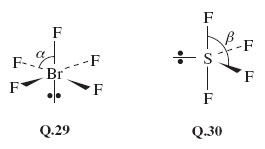## Question 21

Predict the shape and bond angle of (i) the sulfur dioxide molecule, SO2 (central atom S), and (ii) the shape of the molecule XeOF4 (central atom Xe).

• (i) Sulfur has six valence electrons, and four are used up in forming two double bonds to oxygen. This leaves one non-bonded pair, giving a total of three repulsion axes. We predict a V-shaped molecule (Structure Q.31), with a bond angle close to 120°. The experimental value is 119.5°.

•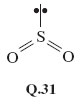• (ii) In XeOF4, two of the eight xenon electrons are involved in the double bond to oxygen, and four in the Xe—F bonds, leaving one non-bonded pair. There are therefore six repulsion axes, and with four electrons assigned to the Xe—O bond, we might expect the repulsions between this bond and the non-bonded pair to be the greatest. The structure that minimises this repulsion is the square-pyramidal Q.32, and this is confirmed by experiment.

•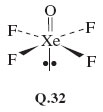## Question 22

For a compound of the typical elements, the brown gas nitrogen dioxide, NO2, is unusual in that its molecule contains an odd number of electrons. Consequently, when applying VSEPR theory to it, a repulsion axis consisting of a single electron is the result. Use VSEPR theory to predict the shape and likely bond angle of NO2 by assessing the repulsive effect that this single-electron repulsion axis might have.